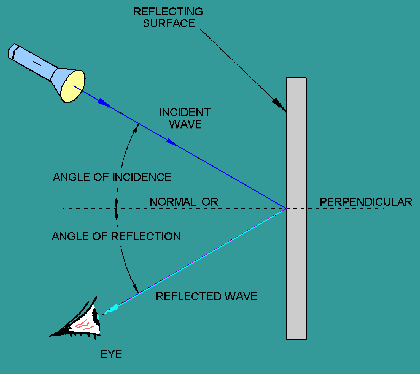Custom SearchReflected waves are simply those waves that are neither transmitted nor absorbed, but are reflected from the surface of the medium they encounter. When a wave approaches a reflecting surface, such as a mirror, the wave that strikes the surface is called the incident wave, and the one that bounces back is called the reflected wave (refer to figure 2-4). An imaginary line perpendicular to the point at which the incident wave strikes the reflecting surface is called the normal, or the perpendicular. The angle between the incident wave and the normal is called the angle of incidence. The angle between the reflected wave and the normal is called the angle of reflection. Figure 2-4. - Reflection of a wave.If the surface of the medium contacted by the incident wave is smooth and polished, each reflected wave will be reflected back at the same angle as the incident wave. The path of the wave reflected from the surface forms an angle equal to the one formed by its path in reaching the medium. This conforms to the law of reflection which states: The angle of incidence is equal to the angle of reflection. The amount of incident-wave energy that is reflected from a surface depends on the nature of the surface and the angle at which the wave strikes the surface. The amount of wave energy reflected increases as the angle of incidence increases. The reflection of energy is the greatest when the wave is nearly parallel to the reflecting surface. When the incidence wave is perpendicular to the surface, more of the energy is transmitted into the substance and reflection of energy is at its least. At any incident angle, a mirror reflects almost all of the wave energy, while a dull, black surface reflects very little. Light waves obey the law of reflection. Light travels in a straight line through a substance of uniform density. For example, you can see the straight path of light rays admitted through a narrow slit into a darkened room. The straight path of the beam is made visible by illuminated dust particles suspended in the air. If the light is made to fall onto the surface of a mirror or other reflecting surface, however, the direction of the beam changes sharply. The light can be reflected in almost any direction, depending on the angle with which the mirror is held. Q.6 What is the law of reflection? Q.7 When a wave is reflected from a surface, energy is reflected. When is the reflection of energy the greatest? Q.8 When is the reflection energy the least? Q.9 Light waves obey what law?Privacy Statement - Copyright Information. - Contact Us